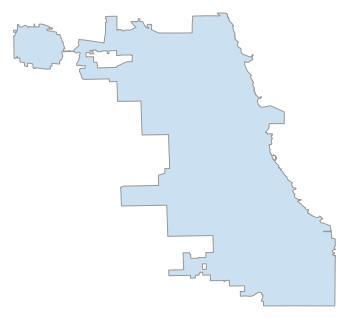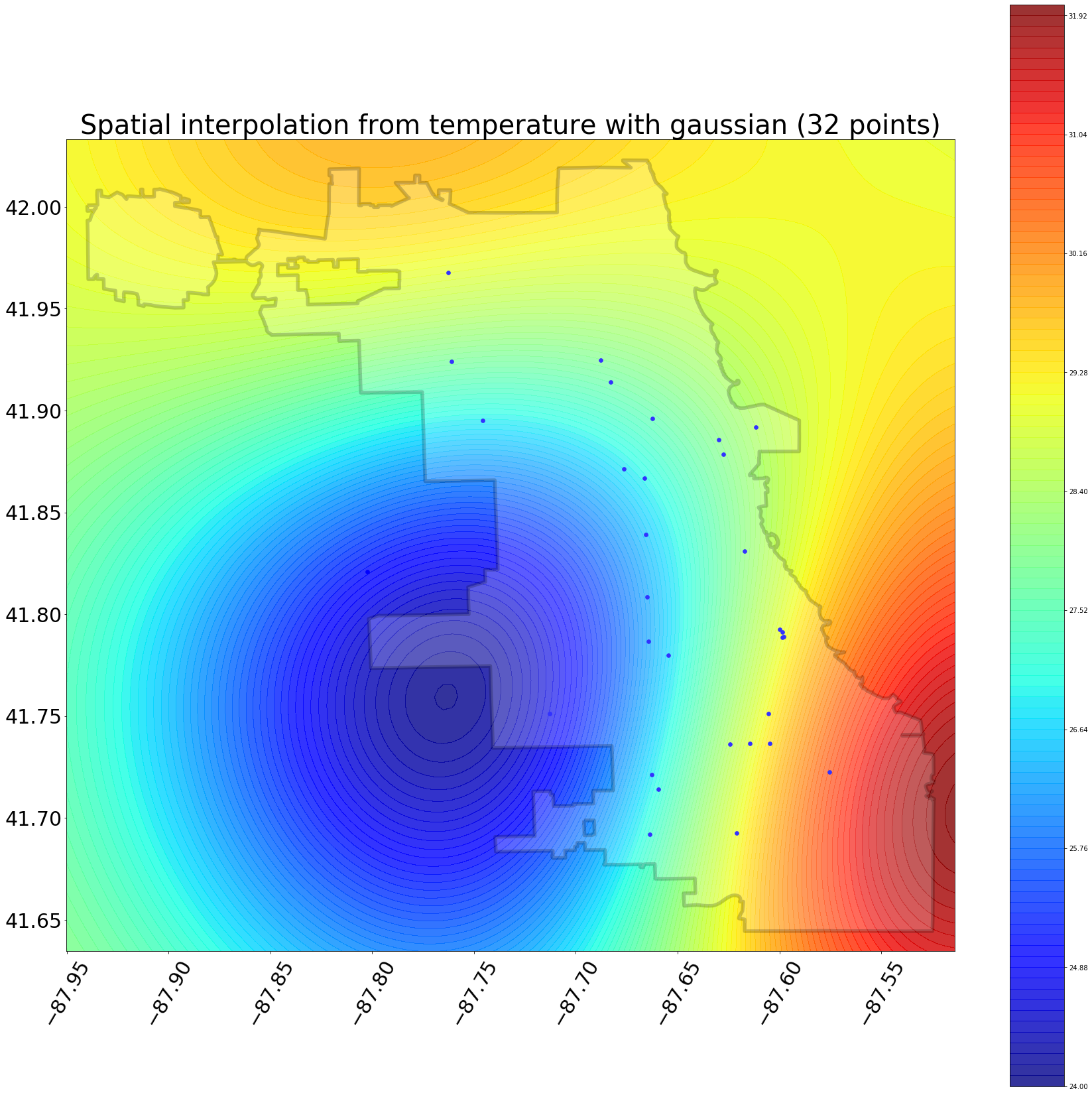# Spatial Interpolation¶

Spatial interpolation is used to predicts values for cells in a raster from a limited number of sample data points around it. We are studying streaming high frequency temperature data in Chicago retrieved from Array of Thing (AoT).

Kriging is a family of estimators used to interpolate spatial data. This family includes ordinary kriging, universal kriging, indicator kriging, co-kriging and others (Taken from Lefohn et al., 2005). The choice of which kriging to use depends on the characteristics of the data and the type of spatial model desired. The most commonly used method is ordinary kriging, which was selected for this study. Reference:

Lefohn, Allen S. ; Knudsen, H. Peter; and Shadwick, Douglas S. 2005. Using Ordinary Kriging to Estimate the Seasonal W126, and N100 24-h Concentrations for the Year 2000 and 2003. A.S.L. & Associates, 111 North Last Chance Gulch Suite 4A Helena , Montana 59601. contractor_2000_2003.pdf

# Notebook Outline¶

## Setup¶

Retrieve the study area of this interactive spatial interpolation jupyter notebook

1) setting the environment, import the library

In :
#!pip install pykrige
import osmnx as ox
%matplotlib inline
ox.config(log_console=True, use_cache=True)
#ox.__version__


2) we choose the Chicago as study area, download the distrct using osmnx, and save the dataset as shapefile

In :
# from some place name, create a GeoDataFrame containing the geometry of the place
city = ox.gdf_from_place('Chicago, IL')
print (city)
# save the retrieved data as a shapefile
ox.save_gdf_shapefile(city)

                                            geometry  \
0  POLYGON ((-87.94010 42.00093, -87.94003 41.998...

place_name  bbox_north  bbox_south  \
0  Chicago, Cook County, Illinois, United States ...    42.02304   41.644531

bbox_east  bbox_west
0 -87.524081 -87.940101


3) plot the Chicago city

In :
fig, ax = ox.plot_shape(city)## Spatial Interpolation¶

The pykrige is a Kriging Toolkit for Python. The code supports 2D and 3D ordinary and universal kriging. Standard variogram models (linear, power, spherical, gaussian, exponential) are built in.

In :
import numpy as np
import pandas as pd
import glob
from pykrige.ok import OrdinaryKriging
from pykrige.kriging_tools import write_asc_grid
import pykrige.kriging_tools as kt
import matplotlib.pyplot as plt
#from mpl_toolkits.basemap import Basemap
from matplotlib.colors import LinearSegmentedColormap
from matplotlib.patches import Path, PathPatch


### Show the points available in Chicago with accurate data¶

Read sensor data in the CSV file, including the sensor ID, latitude, longitude and tempreture.

In :
# uncomment to get from CSV
'sensors.csv',
names=["ID","Lat", "Lon", "Z"])
#data = pd.DataFrame(dd)

Out:
ID Lat Lon Z
0 001e0610ba46 41.878377 -87.627678 28.24
1 001e0610ba13 41.751238 -87.712990 19.83
2 001e0610bc10 41.736314 -87.624179 26.17
3 001e0610ba15 41.722457 -87.575350 45.70
4 001e0610bbe5 41.736495 -87.614529 35.07
5 001e0610ee36 41.751295 -87.605288 36.47
6 001e0610ee5d 41.923996 -87.761072 22.45
8 001e0611441e 41.808594 -87.665048 19.82
9 001e06112e77 41.786756 -87.664343 26.21
10 001e0610f6db 41.791329 -87.598677 22.04
11 001e06113107 41.751142 -87.712990 20.20
12 001e06113ace 41.831070 -87.617298 20.50
13 001e06113a24 41.788979 -87.597995 42.15
14 001e061146cb 41.914094 -87.683022 21.67
15 001e0610f703 41.871480 -87.676440 25.14
16 001e0610e538 41.736593 -87.604759 125.01
17 001e061130f4 41.896157 -87.662391 21.16
18 001e0610ee43 41.788608 -87.598713 19.50
19 001e0610f05c 41.924903 -87.687703 21.61
20 001e0610f732 41.895005 -87.745817 32.03
21 001e06113d20 41.892003 -87.611643 28.30
22 001e06113acb 41.839066 -87.665685 20.11
23 001e061146ba 41.967590 -87.762570 40.60
24 001e0611536c 41.885750 -87.629690 42.80
25 001e061184a3 41.714021 -87.659612 31.46
26 001e06117b44 41.721301 -87.662630 21.35
27 001e061183f5 41.692703 -87.621020 21.99
28 001e061182a7 41.691803 -87.663723 21.62
29 001e06118509 41.779744 -87.654487 20.88
30 001e06118295 41.820972 -87.802435 20.55
31 001e061144be 41.792543 -87.600008 20.41

2） Data processing part, if the tempreture is greater than 45, then set the data be 45

In :
lons=np.array(data['Lon'])
lats=np.array(data['Lat'])
zdata=np.array(data['Z'])
print (zdata)

#If some data are greate than 50, then
for r in range(len(zdata)):
if zdata[r]>50:
zdata[r] = 45

print (zdata)

[ 28.24  19.83  26.17  45.7   35.07  36.47  22.45 125.01  19.82  26.21
22.04  20.2   20.5   42.15  21.67  25.14 125.01  21.16  19.5   21.61
32.03  28.3   20.11  40.6   42.8   31.46  21.35  21.99  21.62  20.88
20.55  20.41]
[28.24 19.83 26.17 45.7  35.07 36.47 22.45 45.   19.82 26.21 22.04 20.2
20.5  42.15 21.67 25.14 45.   21.16 19.5  21.61 32.03 28.3  20.11 40.6
42.8  31.46 21.35 21.99 21.62 20.88 20.55 20.41]


### Use ordinary kriging to do the spatial interpolation¶

In order to run spatial interpolation, we should define the boundary for the Chicago. Get the bounday value from the shapefile.

In :
import geopandas as gpd
Chicago_Boundary_Shapefile = './data/il-chicago/il-chicago.shp'

# get the boundary of Chicago
xmin, ymin, xmax, ymax = boundary.total_bounds


### Generate the grids for longitude and lantitude, the number of bins is 100¶

In :
xmin = xmin-0.01
xmax = xmax+0.01

ymin = ymin-0.01
ymax = ymax+0.01

grid_lon = np.linspace(xmin, xmax, 100)
grid_lat = np.linspace(ymin, ymax, 100)


### Run the OrdinaryKriging method, the variogram_model is gaussian¶

In :
OK = OrdinaryKriging(lons, lats, zdata, variogram_model='gaussian', verbose=True, enable_plotting=False,nlags=20)
z1, ss1 = OK.execute('grid', grid_lon, grid_lat)
print (z1)

Adjusting data for anisotropy...
Initializing variogram model...
Coordinates type: 'euclidean'

Using 'gaussian' Variogram Model
Partial Sill: 23.13242836937993
Full Sill: 93.76725570798001
Range: 0.3088207463280863
Nugget: 70.63482733860009

Calculating statistics on variogram model fit...
Executing Ordinary Kriging...

[[27.92963843313738 27.8858039970517 27.840219117484956 ...
31.386443563996654 31.40455626777043 31.415938741488716]
[27.905896385139272 27.860683189061522 27.81366895016103 ...
31.44165040499307 31.460519582840632 31.47249374835975]
[27.88211218938647 27.835521908251973 27.787080234206595 ...
31.49439048187477 31.514039150198606 31.52663138120343]
...
[29.130760923267935 29.155208360026453 29.180115169970442 ...
29.049409504791104 29.041974883402496 29.034401750480836]
[29.135870577895176 29.160612631119065 29.185827333210476 ...
29.039409247482 29.03163868914854 29.023773683305]
[29.13975307065343 29.164724932520965 29.190180905287033 ...
29.029451254242556 29.021385177488398 29.0132656101344]]


## Plot the spatial interpolation result with ordinary kriging using 'gaussian' variogram model¶

Generate the result and the legend

In :
xintrp, yintrp = np.meshgrid(grid_lon, grid_lat)
fig, ax = plt.subplots(figsize=(30,30))

#ax.scatter(lons, lats, s=len(lons), label='Input data')
boundarygeom = boundary.geometry

contour = plt.contourf(xintrp, yintrp, z1,len(z1),cmap=plt.cm.jet,alpha = 0.8)

plt.colorbar(contour)

boundary.plot(ax=ax, color='white', alpha = 0.2, linewidth=5.5, edgecolor='black', zorder = 5)

npts = len(lons)

plt.scatter(lons, lats,marker='o',c='b',s=npts)

#plt.xlim(xmin,xmax)
#plt.ylim(ymin,ymax)

plt.xticks(fontsize = 30, rotation=60)
plt.yticks(fontsize = 30)

#Tempreture
plt.title('Spatial interpolation from temperature with gaussian (%d points)' % npts,fontsize = 40)
plt.show()
#ax.plot(grid_lon, grid_lat, label='Predicted values')### Run the OrdinaryKriging method, the variogram_model is gaussian¶

In :
OK = OrdinaryKriging(lons, lats, zdata, variogram_model='linear', verbose=True, enable_plotting=False,nlags=20)
z2, ss1 = OK.execute('grid', grid_lon, grid_lat)
#print (z2)

Adjusting data for anisotropy...
Initializing variogram model...
Coordinates type: 'euclidean'

Using 'linear' Variogram Model
Slope: 78.08683547092697
Nugget: 69.86456553372653

Calculating statistics on variogram model fit...
Executing Ordinary Kriging...



### Plot the spatial interpolation result with ordinary kriging using 'linear' variogram model¶

Generate the result and the legend

In :
xintrp, yintrp = np.meshgrid(grid_lon, grid_lat)
fig, ax = plt.subplots(figsize=(30,30))

#ax.scatter(lons, lats, s=len(lons), label='Input data')
boundarygeom = boundary.geometry

contour = plt.contourf(xintrp, yintrp, z2,len(z2),cmap=plt.cm.jet,alpha = 0.8)

plt.colorbar(contour)

boundary.plot(ax=ax, color='white', alpha = 0.2, linewidth=5.5, edgecolor='black', zorder = 5)

npts = len(lons)

plt.scatter(lons, lats,marker='o',c='b',s=npts)

#plt.xlim(xmin,xmax)
#plt.ylim(ymin,ymax)

plt.xticks(fontsize = 30, rotation=60)
plt.yticks(fontsize = 30)

#Tempreture
plt.title('Spatial interpolation from temperature with linear function (%d points)' % npts,fontsize = 40)
plt.show()
#ax.plot(grid_lon, grid_lat, label='Predicted values')